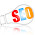## Saturday, June 21, 2014

### String around the Earth circumference Puzzle. If add some length how much hight it rises?

The Puzzle:

The circumference of the Earth is approximately 40,000 km. There is a string circles the Earth, touching the ground at all locations.
Now if you add just 10 meters to its length, How far will it rise above the ground.

I know your guess would be that it will still graze the surface of earth. The raise would be infinitesimally small. But think again that could a fly,
an ant, or a man squeeze underneath it.

Solution:
Let p be Pi=3.14159
Use the formula Circumference = 2 × p × R

Before Circumference = 2 × p × R
After Circumference + 10m = 2 × p × (R + Gap)

Subtracting the two:
10m = 2 × p × Gap

So, the Gap = 10m / (2 × p) = 1.6m approximately

So, a man could fit under it easily.

Voila!! This is amazing discovery.

1.2.3.4.